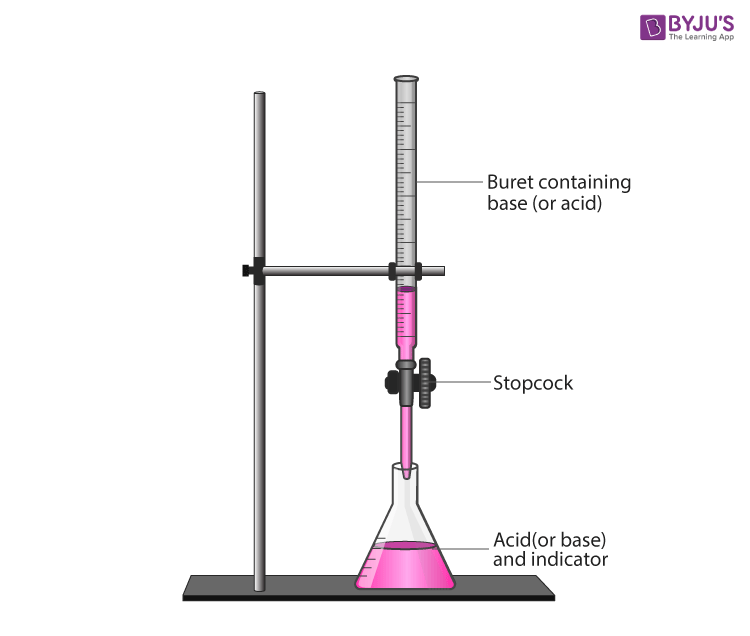Checkout JEE MAINS 2022 Question Paper Analysis : Checkout JEE MAINS 2022 Question Paper Analysis :

# Study The pH Change In The Titration Of A Strong Base Using Universal Indicator

Titration is a process of measuring the volume of a solution of one reactant needed to react completely with a measured amount of another reactant. The point of change in the colour of the solution to which a universal indicator is added determines the point of equivalence of the solution. As the titration takes place, there is a slow change in the pH in the initial stage while approaching the equivalence point, there is a very rapid change in the pH of the solution.

## Aim:

To Learn the pH change in the titration of a strong base using universal indicator

## Materials Required:

Burette, two beakers of 250 mL, 100 mL Conical flask, Dropper, pH charts for reference, 25 mL Hydrochloric acid (0.1 M), 50 mL Sodium Hydroxide solution (0.1 M), Universal indicator 2-3 drops.

## Experimental setup## The Procedure of Titration:

1. Fill 100 mL conical flask with 25 mL hydrochloric acid solution (0.1 M).
2. Fill (0.1 M) sodium hydroxide solution in the burette.
3. Add five drops of universal indicator solution into a conical flask.
4. As indicated in the table below. Drop the solution from the burette into the conical flask and shake the flask.
5. Watch the content of the flask after adding each drop of sodium hydroxide solution.
6. Note the colour of the solution in the conical flask each time you add the solution from the burette and find out the pH by comparing its colour with the pH chart.
7. In the table provided below make a note of your observations.
8. Plot a graph of pH V/s total volume of NaOH from the burette added.

## Observation

Note of pH change observed during the process of neutralization of 25 mL of 0.1 M HCl with 0.1 M NaOH solution

 sl.no Amount of NaOH added in lots (mL) Amount of NaOH added to the solution in flask (mL) pH 1 0 0 2 12.5 12.5 3 10.0 22.5 4 2.3 24.8 5 0.1 24.9 6 0.1 25.0 7 0.1 25.1 8 0.1 25.2 9 0.1 25.3 10 0.1 25.4 11 0.5 25.9

## Precautions to be taken during the experiment:

Take the strong acid and strong base of the same concentration.

Use a minimal quantity of indicator.

## Viva Voice:

1.What is titrate?

The solution which has to be titrated is known as a titrate and is generally taken in a conical flask.

2. What is a titrant?

The solution using which titration is carried out is known as a titrant and is generally taken in a burette flask.

3. What trend of pH change will you observe in the neutralization of strong acid with a strong base?

The endpoint or equivalence point shall be 7.

4. What is the pattern of pH change for neutralization of weak acid (acetic acid) with a strong base (sodium hydroxide) you observe?

There is a sudden change in the pH value at the end of the reaction and it depends on the salt formed.

5.What is the pH range in which the indicator gives a change in colour if the HCl is to be neutralized by NaOH?

Refer the graph. Using titration curve we can find that around the pH range of 7 colour change is witnessed.

Take up a quiz on study-the-ph-change-in-the-titration-of-a-strong-base-using-universal-indicator-experiment